# Using Design of Experiments to Optimize Subwoofer Set-Up

Discussion in 'Audio Science' started by Puma Cat, Nov 18, 2023.

1. ### Puma CatFriend

Pyrate Contributor
Joined:
Sep 7, 2017
766
Trophy Points:
93
Location:
East Bay, CA
Hi Guys,
I thought I'd a share a robust, real-world experimental, statistically-based technique called Design of Experiments to optimize setup of a REL sub into my 2-channel stereo system.

For those interested, first I'm posting an introduction to Design of Experiments here:
_________________

When I started to look at how to effectively integrate my REL R-305 subwoofer with a pair of "mains" speakers using a statistical approach called Design of Experiments to optimize integration of subwoofers for a given room with a set of "mains" speakers.

Description
Design of Experiments (DOE) is a powerful tool that can be used in a variety of experimental situations. DOE allows for multiple input factors to be manipulated determining their effect on a desired output (response). By manipulating multiple inputs at the same time, DOE can identify important interactions that may be missed when experimenting with one factor at a time. All possible combinations can be investigated (full factorial) or only a portion of the possible combinations (fractional factorial). Fractional factorials will not be discussed here.

When to Use DOE
Use DOE when more than one input factor is suspected of influencing an output. For example, it may be desirable to understand the effect of temperature and pressure on the strength of a glue bond.

DOE can also be used to confirm suspected input/output relationships and to develop a predictive equation suitable for performing what-if analysis.

DOE Procedure
Acquire a full understanding of the inputs and outputs being investigated. A process flow diagram or process map can be helpful. Utilize subject matter experts as necessary.

Determine the appropriate measure for the output. A variable measure is preferable. Attribute measures (pass/fail) should be avoided. Ensure the measurement system is stable and repeatable.

Create a design matrix for the factors being investigated. The design matrix will show all possible combinations of high and low levels for each input factor. These high and low levels can be generically coded as +1 and -1. For example, a 2 factor experiment will require 4 experimental runs: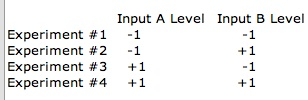Note: The required number of experimental runs can be calculated using the formula 2n where n is the number of factors.

For each input, determine the extreme but realistic high and low levels you wish to investigate. In some cases the extreme levels may be beyond what is currently in use. The extreme levels selected should be realistic, not absurd. For example:

Enter the factors and levels for the experiment into the design matrix. Perform each experiment and record the results. For example: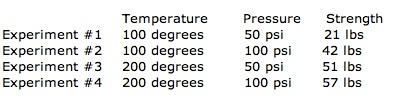Calculate the effect of a factor by averaging the data collected at the low level and subtracting it from the average of the data collected at the high level. For example:

Effect of Temperature on strength:
(51 + 57)/2 - (21 + 42)/2 = 22.5 lbs

Effect of Pressure on strength:
(42 + 57)/2 - (21 + 51)/2 = 13.5 lbs

The interaction between two factors can be calculated in the same fashion. First, the design matrix must be amended to show the high and low levels of the interaction. The levels are calculated by multiplying the coded levels for the input factors acting in the interaction. For example: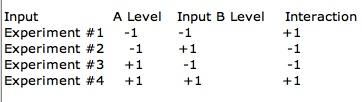Calculate the effect of the interaction as before.

Effect of the interaction on strength:
(21 + 57)/2 - (42 + 51)/2 = -7.5 lbs

The experimental data can be plotted in a 3D Bar Chart.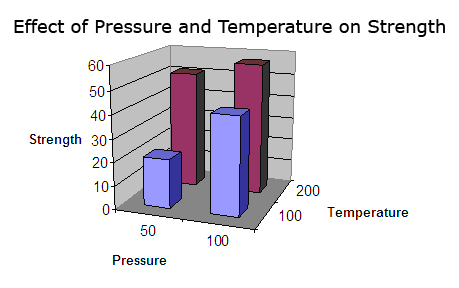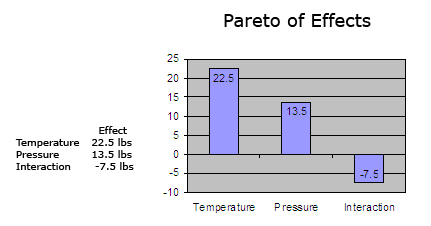This simple-minded example above shows that there is an interaction between temperature and pressure in the strength of the glue bond. This is one feature of DOEs that is particularly useful when looking at the effect of a number of factors and their effect on the critical functional response.

Now that that is out of the way as intro, let's look at the specific experiment I had in mind in the next post.

The experiment I was trying to reproduce was the one based on the well-known "Harman curve" showing that listeners perceived a room as having flat room reponse when the measured frequency actually looked more like this. This is because our hearing is not linear at lower frequencies.

Now, one could debate whether the Harman curve is exactly the right curve on which to base this model, but it was a well-characterized "orthogonal reference" as we say in science, and as such, it was the one I decided to use for this experiment. Please keep in mind this was just an experiment I did for fun while recovering from a back operation. What can I say...this is how scientists like myself are "wired".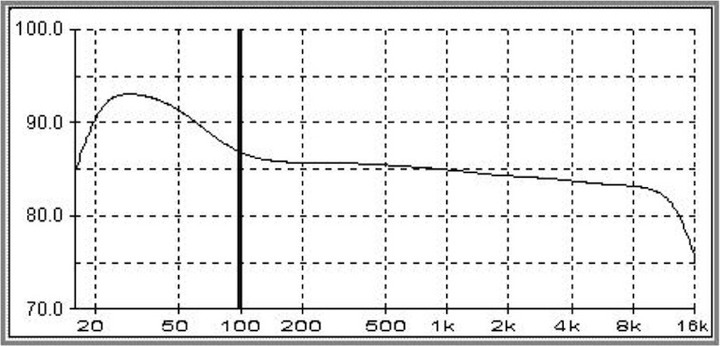Given this, I set out to to perform a "DOE" to see if I could determine the optimal settings of speakers, REL sub settings and other factors, like grilles on or off, speaker toe-in, port plugs, etc. that would optimize the in-room response.

With that in mind, I set out to see what I could do with my set-up to obtain, as close as possible, the Harman response curve via DOE.

System used at the time was: Conrad-Johnson Premier 17LS Preamp, Conrad-Johnson LP70S, Dynaudio Contour S3.4 speakers, REL R-305, M-Audio MobilePre USB mike preamp, Dayton NIST-traceable calibrated measurement microphone with calibration file, and Room EQ Wizard Software. The Room EQ Wizard software generates and simultaneously measures the frequency sweep from 10 Hz to 20,000 Hz.

The desired responses were to maximize 20 and 50 Hz repsonse in dB, and minimize the 150 and 500 Hz responses. These 150 and 500 Hz responses were nodes that I wanted to minimize, if possible.

An example trace as my starting point for reference is shown. The purple trace is where I started with the sub in, the brown trace with the sub out, before optimizing things using the DOE approach. The following is the real-time, IN-ROOM measured response for the right speaker: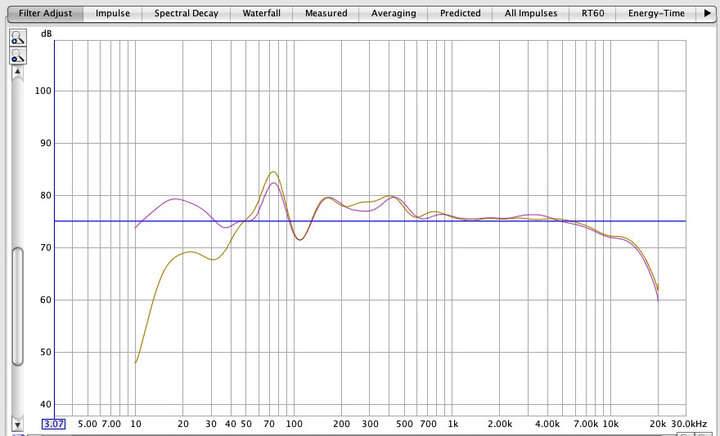The goal here was to maximize the 20-50Hz node to range, while smothing the 70, and minimizing the 105, 155 and 500 Hz node peaks, so as to emulate the Harman graph.

The factors I used for the DOE were sub gain (as clicks up from zero), sub crossover, likewise clicks up from zero, plug or no plug in the speaker reflex port. So, four responses being measured as the result of 3 factors at two different levels (low, high as in the examples above shown).

Setting up a full-factorial DOE in JMP (a stastical package), here are the experimental matrix I ran per JMP's output for the experimental design and the ACTUAL measurements for each frequency (as measured in dB by room Eq Wizard).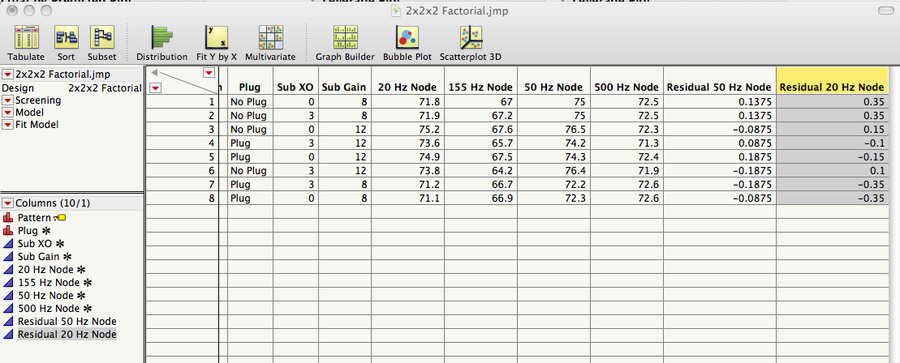The next post will be the analysis of the data...

•Like x 4
•Epic x 4
•heart x 1
• List
Last edited: Nov 19, 2023
2. ### Puma CatFriend

Pyrate Contributor
Joined:
Sep 7, 2017
766
Trophy Points:
93
Location:
East Bay, CA
Here is the result of analyzing the 20 Hz response of the DOE in JMP....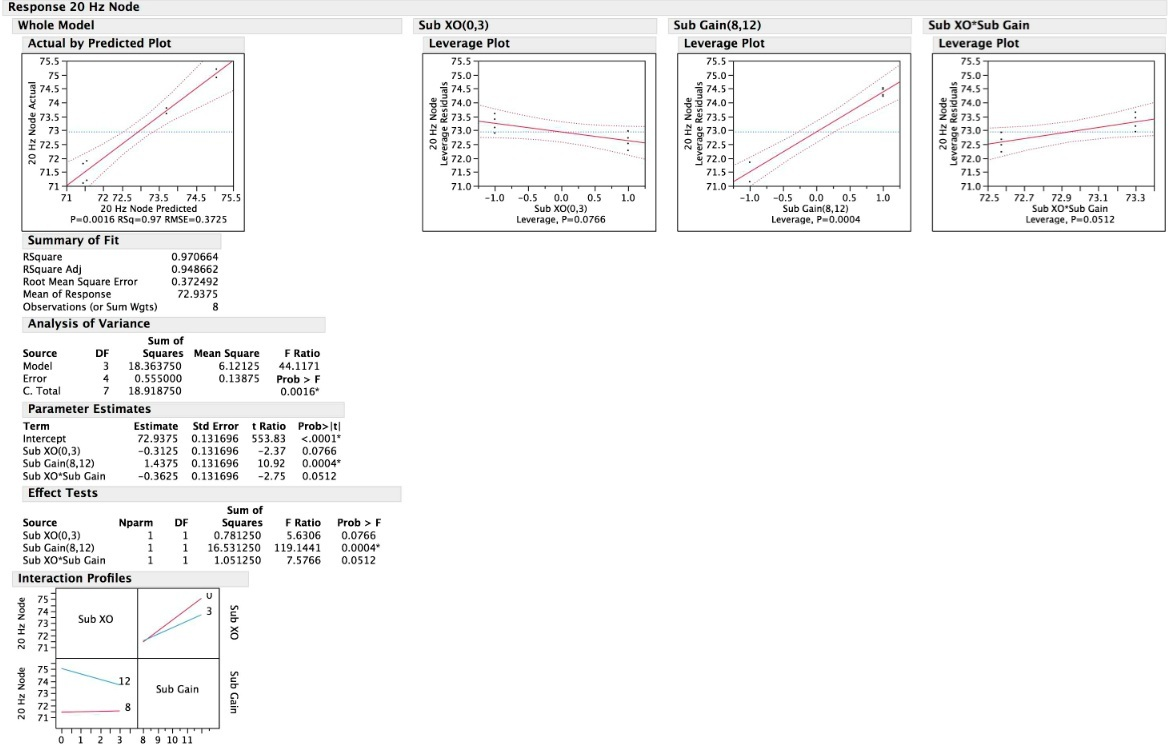We can see from the actual by predicted plot that the R^2 and R^2-Adjusted are strongly correlated at 0.97 and 0.94, respectively, indicating that our results fit our model pretty well. Also the leverage plots (the factors affecting the response (20Hz output), show that Sub gain has a large effect, and quite possibly that the interaction of sub gain and sub crossover may have an effect, as the p-value is just barely above p>-.05, which tends to suggest there may be a significant effect if we gather more data or relax our confidence level from 95% to 90%. In, fact, if we relax our alpha from 5% (the chance we are willing to accept that we are wrong) to 10%, then the Sub crossover point becomes significant. In addition, our Analysis of Variance with probability of F > 0.0016 tells us that that the "chances" this result occurred from the null hypothesis being true, i.e., that the model does not predict sub 20 Hz performance is very low. We can there therefore reject the Null Hypothesis that the sub does not have an effect, and accept the Alternate Hypothesis that the sub drives the LF response in the room with 99.84% statistical confdence.

The interaction profile plots also suggest that there is a likely interaction between sub XO setting and sub gain, and the plot lines are not perfectly parallel, but appear to intersect.

Looking at the Prediction Profiler from JMP, comparing Sub gain with Sub XO, we can see that the maximum 20 Hz response is obtained by the Sub gain at 12 clicks up, and sub set at zero clicks, and we could expect a level of 75.1875 dB.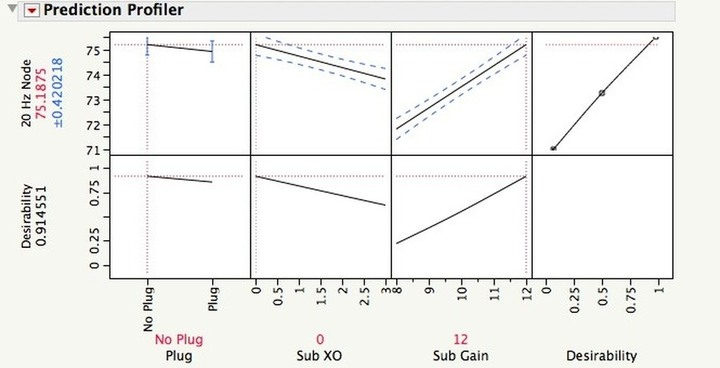JMP is also cool because it will show you a 3-D image of two factors at one time as they affect the desired response in a 3-D cube, in this case, the 20 Hz response. As you can see, the upper left corner of the rectangle shows, which is max 20 Hz response is at a sub crossover of zero, and a gain setting of 12.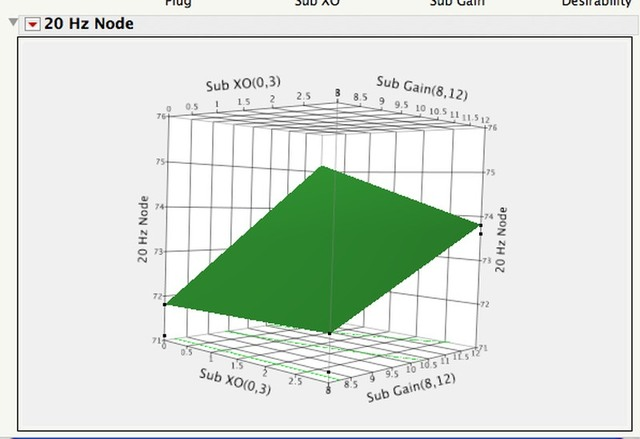These analyses show how you can utitlize DOE to predict with good confidence the response from setting the factors (crossover point and gain) to give the desired response at 20 Hz.

I used this approach then to measure the BEFORE and AFTER responses, in this graph, BEFORE is BLUE and AFTER is GREEN. Note how much smoother the overall frequency response is for the green trace. Compare the green trace to the yellow trace in the graph above before optimizing the REL set-up prior to the DOE. You can see it's much smoother than the original response, and doesn't have the influence of the room modes on the FR.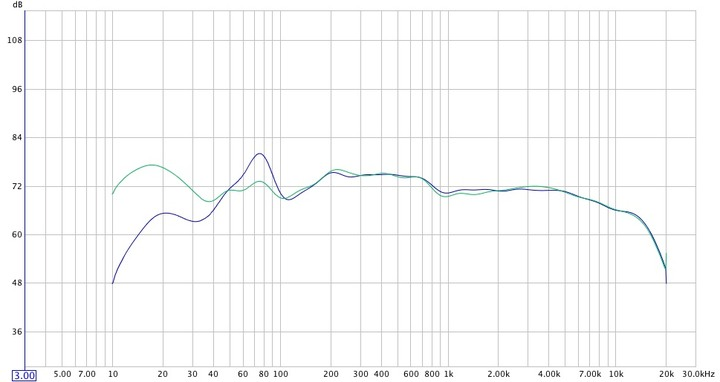•Epic x 6
•Like x 1
• List
Last edited: Nov 19, 2023
3. ### Puma CatFriend

Pyrate Contributor
Joined:
Sep 7, 2017
766
Trophy Points:
93
Location:
East Bay, CA
The nice thing about using DOEs is, it's takes the guesswork out of optimizing a system. You literally obtain F-statistics and p-values that specify how accurately the DOE model "explains" the entirety of the measurement data for your system under experimentation and...how it "fits" into the real-world response. In statistics, we generally set our confidence level at 95% confidence, which means the alpha-risk (which the chance you're willing to reject the null hypothesis when it is in fact true, i.e., accept the risk of the model "being wrong") is set to 0.05 (5%).

What this means, basically, is that any DOE model with a p-value < 0.05 is considered to be statistically significant with respect to having 95% confidence or greater. In the case of this DOE model for integrating the REL sub, our p-value is 0.0016, which means we have 99.84% confidence that this model explains the actual data observed. And...that's pretty darn good statistical confidence.

DOEs are incredibly powerful tools and you can use it for many audio applications. For example, you could use it to optimize speaker placement, or cartridge alignment and setup, for example.

•Like x 1
• List
4. ### Puma CatFriend

Pyrate Contributor
Joined:
Sep 7, 2017
766
Trophy Points:
93
Location:
East Bay, CA
One of the really powerful things about DOEs, is you can build a good model, it can be use to show the "response" of the measurement(s) under study.

Now, most folks are just taught in school about fairly simple approaches to characterizing a response, e.g. using a linear regression, where the output is plotted as straight line based on the levels of the input factors.

An example are the plots from the Sub's DOE showing the influence of Sub Crossover (XO) and Gain on the model. These plots are simple linear regression plots. Basically, you turn Knob "X" and you get response "Y" changing in a linear proportion to the change in X.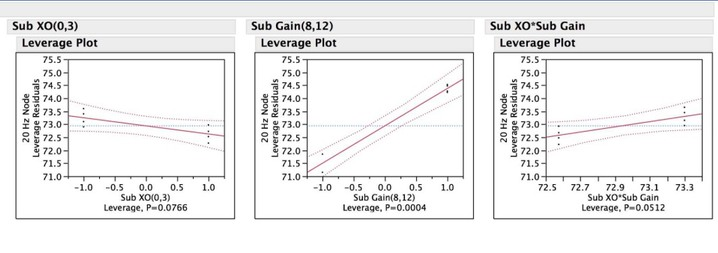But...DOEs are much more powerful than that, and can shown the nature of....interactions. For example, take the Time*Temp interaction when doing something as simple as...baking cookies. It's not Time and it's not Temp that produces a properly baked cookie, it's the INTERACTION of Time*Temp that produces a properly-baked coookie. This is important to understand because University typically does not teach or demonstrate the influences of INTERACTIONS to students when teaching science or engineering. Instead they teach OFAT: One Factor At a Time. Unfortunatly, often times the real world simply does not work in as simplistic a manner as OFAT.

Here's an example of what DOEs can also do: this is a response surface plot from the Sub DOE above depicting the INTERACTION between sub GAIN and sub CROSSOVER at the 155 Hz room mode. As is obvious, the fuctional response is not a simple staight line e.g. in a linear regression. It's 3-dimensional shape that has curvature and complexity to it.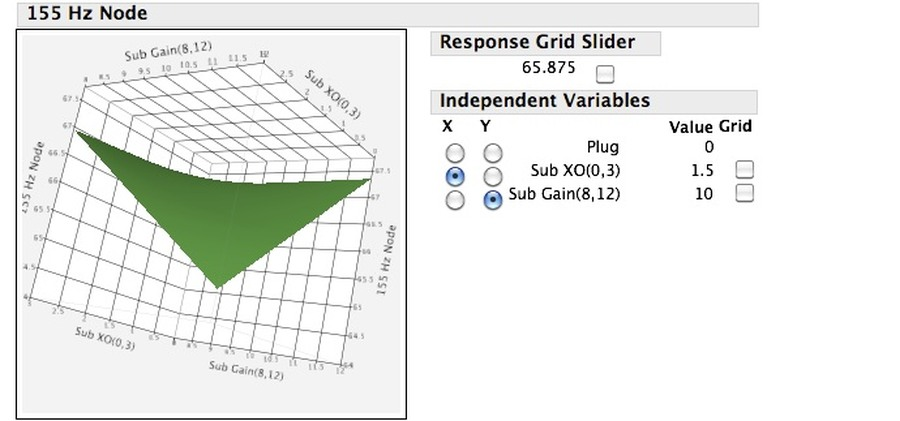And..if you rotate the response surface cube around, you see more of the actual shape of the 3-dimensional response curve of the 155 Hz room mode.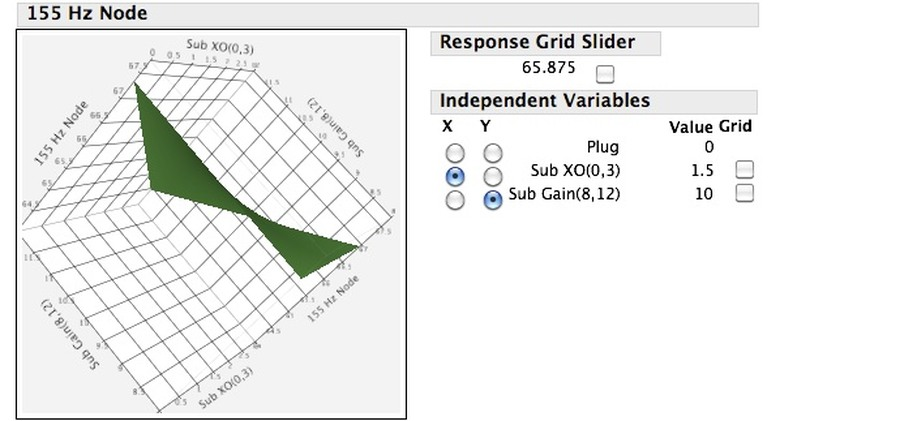The operative point here being is to really fully characterize and thus, understand, what's going on your room with your components and speakers interacting with the room itself (remember, Danny Ritchie will tell you that the room itself is one of the most important factors), tools such as DOEs provide INSIGHT into the settings you'll need to obtain the best, real-world response.

Don'tcha just love...(real) SCIENCE?•Like x 3
• List
5. ### famish99Friend

Pyrate
Joined:
Dec 26, 2017
1,693
Trophy Points:
93
Location:
Austin, TX
My follow up question to this, would be how would you use DOE to run experiments where you're chasing a qualitative rather than quantitative end result? Would you be able to regress against blind trial p-values or would it have to be something else?

It would be interesting to see how this can be leveraged to optimize a subjective outcome.

6. ### Puma CatFriend

Pyrate Contributor
Joined:
Sep 7, 2017
766
Trophy Points:
93
Location:
East Bay, CA
It's a very good question.

You could potentially use DOEs in situations where a "qualitatively-assigned" number or score can be applied, per se, but for that, you'd need very consistent, "scoring" for assigning the numerical value and you'd have to perform a statistically-valid MSA (Measurement System Analysis, also based on ANOVA, BTW) prior to conducting the DOE to ensure repeatibility and reproducibility (which, BTW, are not the same thing) by the Operators making the "qualitative" measurement assessment. But, a simple P/F asssessment is not gonna work.

Basically, the answer is that DOE is (generally) only applicable when you have a "continuous data" measure, i.e., where "decimal numbers" make sense. And, of course you still need a statistically validated measurement system for continuous data, so you still need to do the MSA before doing your DOEs.

•Like x 1
• List